# Python Decision-Making Statements

Decision-making statements in Python help control the program based on a required or given condition. These statements are all used to determine the order of execution of other specified statements in the program.

## Types of Decision-Making Statements

The four main categories of decision-making statements are as follows.

All these decision-making statements are described with examples and in detail in one separate tutorial. Therefore, all the links go to a single tutorial page.

## Python Decision-Making Examples

As all the decision-making statements are described in separate tutorials, Therefore, here we only see the basic example program based on those statements. Let's create a simple example that demonstrates a decision-making statement in Python:

```print("Enter a Number: ")
val = int(input())

if val>0:
print("You've entered a number, greater than 0.")```

The initial output produced by the above program is shown in the snapshot given below: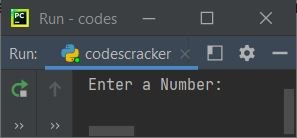Now enter a number, say 14, and press the ENTER key to see the output as shown in the snapshot given below: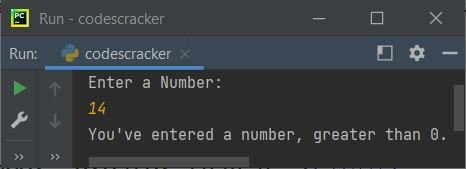In the above program, the first statement is:

`print("Enter a Number: ")`

prints the message "Enter a Number" on the output screen. And the second statement is:

`val = int(input())`

receives the entered number using "input()," and the value gets converted into an integer type using "int()." Finally, the value gets initialized in the "val" variable. So if the user enters 14 as input, then "val=14." Now the condition of "if" gets evaluated. That is, the condition "val>0" or "14>0" evaluates to be true, therefore program flow goes inside the "if"'s body. And the statement:

`print("You've entered a number, greater than 0.")`

prints "You've entered a number, greater than 0."  on the output screen. That's it. Now let's modify the above program, which uses two decision-making statements:

```print("Enter a Number: ")
val = int(input())

if val>0:
print("You've entered a number, greater than 0.")
else:
print("You've entered a number, equals to 0 or less.")```

Here is a sample run with -20 user input: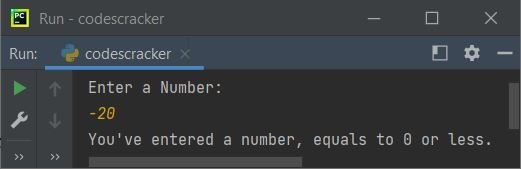In the above program, if the condition val>0 evaluates to be true, then program flow goes to if's body; otherwise, it goes to else's body. Now here is the third program created, which uses three decision-making statements:

```print("Enter a Number: ")
val = int(input())

if val>0:
print("You've entered a number, greater than 0.")
elif val==0:
print("You've entered 0.")
else:
print("You've entered a number, less than 0.")```

Here's an example of a run with user input 0: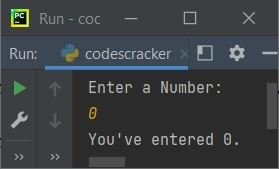In the above program, first the condition of the "if" gets evaluated; if the condition evaluates to be false, then the condition of the "elif" gets evaluated.

If the condition of the "if" evaluates to true, then program flow goes to its body. Otherwise, if the condition of the "elif" evaluates to true, then program flow goes into its body. And if both conditions evaluate to false, then the program flow goes to the "else" body.

Note: You can provide as many conditions as possible by using multiple "elif," lying between "if" and "else."

Here is the last program that uses all the decision-making statements, including nested if:

```print("Enter a Number: ", end="")
val = int(input())

if val>0:
if val<10000:
print("The number is less than 10000.")
else:
print("The number is a big number.")
elif val==0:
print("You've entered 0.")
else:
print("The number is a negative number.")
if val>-100:
print("The number is greater than -100.")```

Here is its sample output with user input of -40: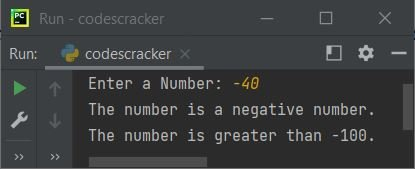#### More Examples

Python Online Test

« Previous Tutorial Next Tutorial »